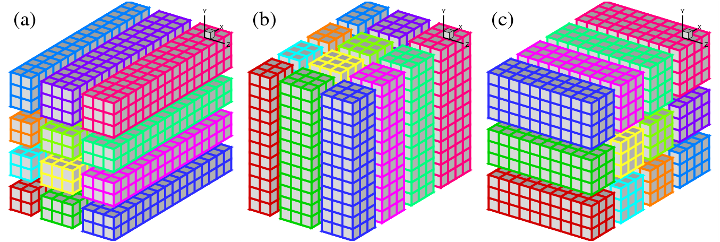Library for 2D pencil decomposition and distributed Fast Fourier Transform

### Using the 1D Decomposition Mode

While 2DECOMP&FFT implements a general-purpose 2D pencil decomposition library, 1D slab decomposition remains an attractive option for certain applications.

• For small-to-medium size applications, which are unlikely to hit the constraint imposed by the decomposition strategy, having simpler and more efficient implementations is often preferable.
• There are applications with algorithms that can not be easily split to multiple 1D operations (for example a Poisson solver using 1D FFT in a homogeneous direction and a 2D multigrid solver in the two remaining directions).
• For applications with multiple levels of parallelism, it may be more convenient to use 1D decomposition for the coarse-grain level data distribution. Then on each slab fine-grain parallelism can be applied (e.g. using OpenMP on shared-memory node).Figure 1: 2D pencil decomposition example using a P_row*P_col=4*3 processor grid:
(a) X-pencil; (b) Y-pencil; (c) Z-pencil.

Fig.1 shows an arbitrary 3D domain partitioned using a 2D processor grid of P_row=4 by P_col=3. Clearly 1D decomposition is just a special case of 2D decomposition with either P_row=1 or P_col=1. In both cases, the communication algorithms can be simplified significantly.Figure 2: 1D slab decomposition of the same domain as in Fig.1
Left: P_row=1; Right: P_col=1.

If P_row=1, state (a) and (b) are identical, as shown in Fig.2 (left); similarly, for P_col=1, state (b) and (c) are identical, shown in Fig.2 (right). So the 1D decomposition can be defined as either slabs in Y and Z or slabs in X and Y. The former is often preferred as better cache efficiency may be achieved by always keeping the X direction in local memory.

When using the FFT library with 1D decomposition, half of the global transpositions can be dropped, resulting in more efficient code. This optimisation was introduced in version 1.1.x of 2DECOMP&FFT.

Finally, note that one can also rely on this arrangement to perform large distributed 2D simulations. For example one option is to define the 2D data sets in an X-Y plane by setting nz=1 and P_col=1 (arrays are still to be declared as 3D to satisfy the programming interface of the library).